Descriptive Statistics

# 11 Geometric Mean

The mean (Arithmetic), median and mode are all measures of the “center” of the data, the “average”. They are all in their own way trying to measure the “common” point within the data, that which is “normal”. In the case of the arithmetic mean this is solved by finding the value from which all points are equal linear distances. We can imagine that all the data values are combined through addition and then distributed back to each data point in equal amounts. The sum of all the values is what is redistributed in equal amounts such that the total sum remains the same.

The geometric mean redistributes not the sum of the values but the product of multiplying all the individual values and then redistributing them in equal portions such that the total product remains the same. This can be seen from the formula for the geometric mean,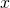: (Pronounced x-tilde)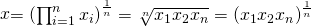where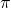is another mathematical operator, that tells us to multiply all the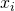numbers in the same way capital Greek sigma tells us to add all thenumbers. Remember that a fractional exponent is calling for the nth root of the number thus an exponent of 1/3 is the cube root of the number.

The geometric mean answers the question, “if all the quantities had the same value, what would that value have to be in order to achieve the same product?” The geometric mean gets its name from the fact that when redistributed in this way the sides form a geometric shape for which all sides have the same length. To see this, take the example of the numbers 10, 51.2 and 8. The geometric mean is the product of multiplying these three numbers together (4,096) and taking the cube root because there are three numbers among which this product is to be distributed. Thus the geometric mean of these three numbers is 16. This describes a cube 16x16x16 and has a volume of 4,096 units.

The geometric mean is relevant in Economics and Finance for dealing with growth: growth of markets, in investment, population and other variables the growth in which there is an interest. Imagine that our box of 4,096 units (perhaps dollars) is the value of an investment after three years and that the investment returns in percents were the three numbers in our example. The geometric mean will provide us with the answer to the question, what is the average rate of return: 16 percent. The arithmetic mean of these three numbers is 23.6 percent. The reason for this difference, 16 versus 23.6, is that the arithmetic mean is additive and thus does not account for the interest on the interest, compound interest, embedded in the investment growth process. The same issue arises when asking for the average rate of growth of a population or sales or market penetration, etc., knowing the annual rates of growth. The formula for the geometric mean rate of return, or any other growth rate, is: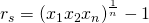Manipulating the formula for the geometric mean can also provide a calculation of the average rate of growth between two periods knowing only the initial valueand the ending value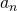and the number of periods,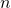. The following formula provides this information: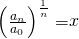Finally, we note that the formula for the geometric mean requires that all numbers be positive, greater than zero. The reason of course is that the root of a negative number is undefined for use outside of mathematical theory. There are ways to avoid this problem however. In the case of rates of return and other simple growth problems we can convert the negative values to meaningful positive equivalent values. Imagine that the annual returns for the past three years are +12%, -8%, and +2%. Using the decimal multiplier equivalents of 1.12, 0.92, and 1.02, allows us to compute a geometric mean of 1.0167. Subtracting 1 from this value gives the geometric mean of +1.67% as a net rate of population growth (or financial return). From this example we can see that the geometric mean provides us with this formula for calculating the geometric (mean) rate of return for a series of annual rates of return: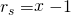where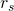is average rate of return andis the geometric mean of the returns during some number of time periods. Note that the length of each time period must be the same.

As a general rule one should convert the percent values to its decimal equivalent multiplier. It is important to recognize that when dealing with percents, the geometric mean of percent values does not equal the geometric mean of the decimal multiplier equivalents and it is the decimal multiplier equivalent geometric mean that is relevant.

### Formula Review

The Geometric Mean:### What is the geometric mean of the data set given? 5, 10, 20

10

What is the geometric mean of the data set given? 9.000, 15.00, 21.00

14.15

What is the geometric mean of the data set given? 7.0, 10.0, 39.2

14

What is the geometric mean of the data set given? 17.00, 10.00, 19.00

14.78

What is the average rate of return for the values that follow? 1.0, 2.0, 1.5

44%

What is the average rate of return for the values that follow? 0.80, 2.0, 5.0

100%

What is the average rate of return for the values that follow? 0.90, 1.1, 1.2

6%

What is the average rate of return for the values that follow? 4.2, 4.3, 4.5

33%

### Homework

An investment grows from ?10,000 to ?22,000 in five years. What is the average rate of return?

17%

An initial investment of ?20,000 grows at a rate of 9% for five years. What is its final value?

?30,772.48

A culture contains 1,300 bacteria. The bacteria grow to 2,000 in 10 hours. What is the rate at which the bacteria grow per hour to the nearest tenth of a percent?

4.4%

An investment of ?3,000 grows at a rate of 5% for one year, then at a rate of 8% for three years. What is the average rate of return to the nearest hundredth of a percent?

7.24%

An investment of ?10,000 goes down to ?9,500 in four years. What is the average return per year to the nearest hundredth of a percent?

-1.27%